Microsoft

# How to Display Top and Bottom N in a Single Visual Using Slicer Dynamically in Power BIIn Power BI, Top N and Bottom N are often displayed using a visual level filter and two visuals, respectively. As a result, we can only show one item at a time on top or bottom N. But, if the situation asks for slicer selection to be used to dynamically change how top and bottom N are shown in a single visual at simultaneously. DAX can help us accomplish this scenario. Let’s look at how we can visualize this situation.

## Steps:

### 1. Create a measure like Total Quantity to display the total number of orders.

Total Order = COUNT(order details[order_id])

### 2. Create a table and generate a series in that table by using the below Dax which will be used for slicer selection.

Slicer = (GENERATESERIES(1,10))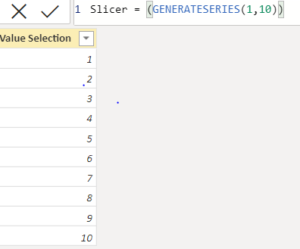### 3. Now create a measure that will be used on the visual level filter to display Top and Bottom N both in a single visual at the same time.

Top Bottom =

Var TopX= SELECTEDVALUE(Slicer[Value Selection])

Var TopRank= RANKX(ALL(pizza_types[name]),CALCULATE(‘Calculations'[Total Order]),,DESC)

Var BottomRank= RANKX(ALL(pizza_types[name]),CALCULATE(‘Calculations'[Total Order]),,ASC)

Var Result=

SWITCH(

TRUE(),

TopRank<=TopX,1,

BottomRank<=TopX,-1,

blank())

return Result

### Explanation: In the above measure

1. In TopX we are taking the values which are there in the column which we created for slicer selection.
2. In TopRank we are giving rank to pizza names and their Total number of orders in Descending order.
3. In BottomRank we are giving rank to pizza names and their Total number of orders in Ascending order.
4. Then in Result, we are putting the Condition that if TopRank is less than or equal to TopX then give the result as 1, and if BottomRank is less than or equal to TopX then give the result as -1 else give blank.
5. Then we are returning the Result.

### 4. Now place the column in the slicer from the table in which we generated the series.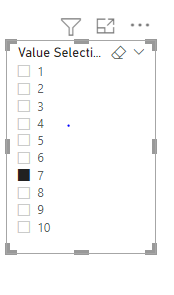### 5. Now take the bar chart on canvas and drag the total orders in the value section and pizza names on X-axis and Place the Top Bottom measure in the Visual level filter, select is not blank, and apply the filter.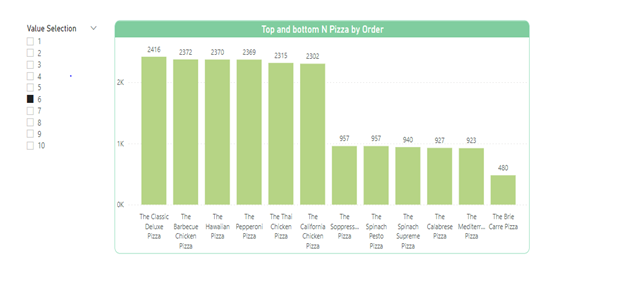### 6. Now we want to display top orders in green and bottom orders in red then we can give conditional formatting to the visual. We need to make a measure and place it in the data color function.

Measure:> Color = SWITCH(TRUE(),

‘Calculations'[Top Bottom]=1,”#43E114″,

[Top Bottom]=-1,”#E13014″)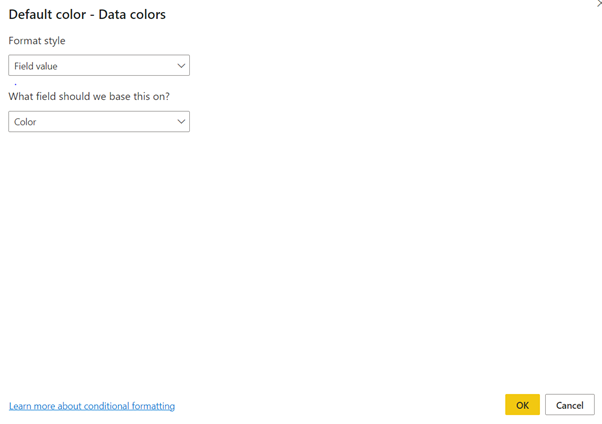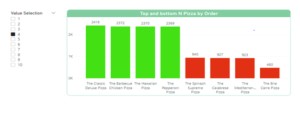That’s how we can display Top and Bottom N both in Single Visual at the same time in Power BI.

## Thoughts on “How to Display Top and Bottom N in a Single Visual Using Slicer Dynamically in Power BI”

1.Suraj Singh Thakur

Nice blog!!! Anshika

This site uses Akismet to reduce spam. Learn how your comment data is processed.

##### Anshika Bajpai

Anshika Bajpai is an Associate Technical Consultant at Perficient Nagpur GDC. She is a Power BI developer having one year of Experience in Power BI. She likes to share her knowledge and is open to suggestions as well.

More from this Author